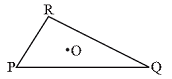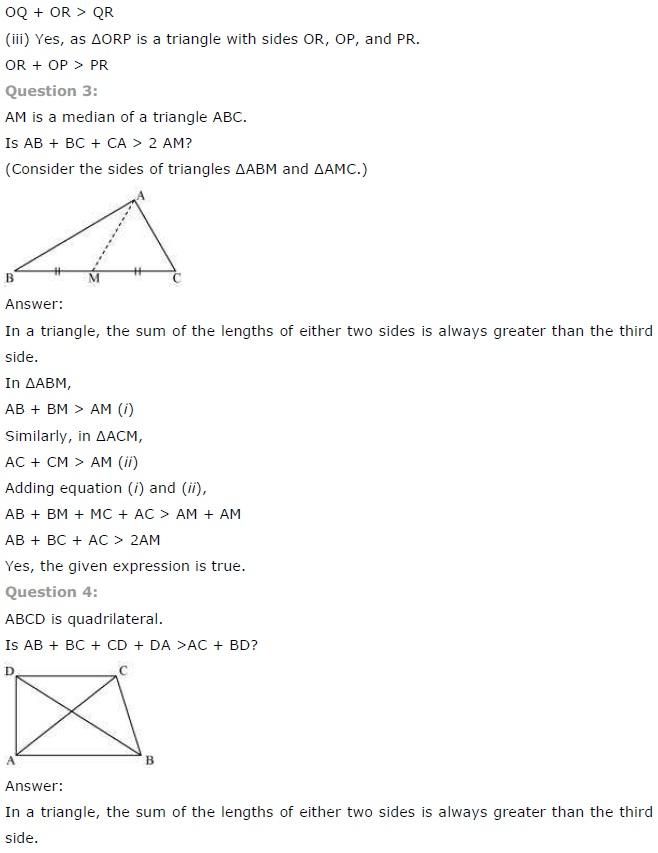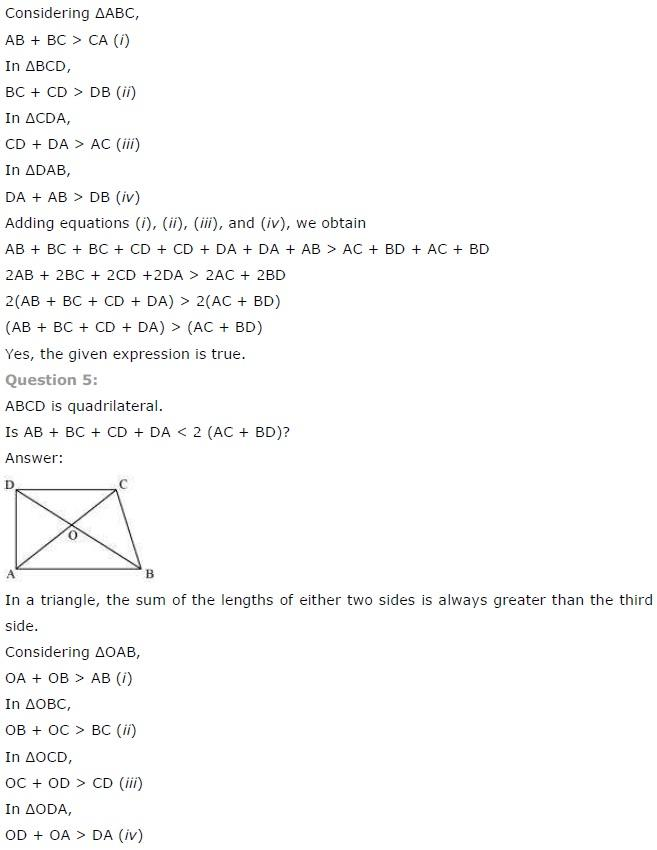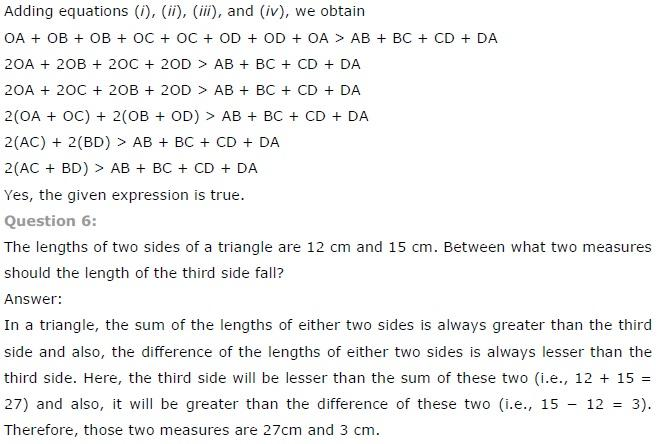# NCERT Solutions class 7 Maths Chapter-6 Exercise 6.4

NCERT Solutions Class-7 Maths chapter-6 Triangle and its properties Exercise-6.4 is prepared by academic team of pw all the questions of NCERT text book are solved step by step with proper and detail solutions explaining each and every questions . For More and additional questions of CBSE class 7 maths you can go to class 7 maths sections. NCERT class 7 Maths Solutions is the best way to enhanced your mathematics skill. And pw practice worksheet & question bank will help you a lot .

## NCERT Solutions class 7 Maths Chapter-6 Triangle and its properties

### Solutions of Chapter Triangle and its properties Exercise-6.4

Question 1:
Is it possible to have a triangle with the following sides?
(i) 2 cm, 3 cm, 5 cm
(ii) 3 cm, 6 cm, 7 cm
(iii) 6 cm, 3 cm, 2 cm

(i) 2 cm, 3 cm, 5 cm
We know that,
In a triangle, the sum of the length of either two sides of the triangle is always greater than the third side
The given sides of triangle in this question are:
2 cm, 3 cm and 5 cm
Now, 2 + 3 + = 5 cm
5 cm = 5 cm
Hence,
The triangle is not possible as the sum of the length of either two sides of the triangle is not greater than the third side

(ii) 3 cm, 6 cm, 7 cm
We know that,
In a triangle, the sum of the length of either two sides of the triangle is always greater than the third side
The given sides of triangle in this question are:
3 cm, 6 cm and 7 cm
Now, 3 + 6 = 9 cm and 9 cm > 7 cm
6 + 7 = 13 cm and, 13 cm > 3 cm
3 + 7 = 10 cm and, 10 cm > 6 cm
Hence,
The triangle is possible as the sum of the length of either two sides of the triangle is greater than the third side

(iii) 6 cm, 3 cm, 2 cm
We know that,
In a triangle,
The sum of the length of either two sides of the triangle is always greater than the third side
Therefore,
The given sides of triangle in this question are:
6 cm, 3 cm and 2 cm
Now,
6 + 3 = 9 cm and 9 cm > 2 cm
3 + 2 = 5 cm But, 5 cm < 6 cm
Hence,
The triangle is not possible as the sum of the length of either two sides of the triangle is not greater than the third side

Question 2:
Take any point O in the interior of a triangle PQR.  Is:
(i) OP + OQ > PQ?
(ii) OQ + OR > QR?
(iii) OR + OP > RP?(i) OP + OQ > PQ?
According to the given condition in the question,
If O is a point in the interior of the given triangle
Then,
Three triangles can be constructed, these are:
∆OPQ, ∆OQR and ∆ORP
We know that,
In a triangle, the sum of the length of either two sides of the triangle is always greater than the third side
Therefore,
∆OPQ is a triangle having sides OP, OQ and PQ
As,
OP + OQ > PQ

(ii) OQ + OR > QR?
According to the given condition in the question,
We have:
If O is a point in the interior of the given triangle
Then,
Three triangles can be constructed, these are:
∆OPQ, ∆OQR and ∆ORP
We know that,
In a triangle,
The sum of the length of either two sides of the triangle is always greater than the third side
Therefore,
∆OQR is a triangle having sides OR, OQ and QR
As,NCERT CLASS 7 MATHEMATICS SOLUTIONS